• 前言： 本文介绍LASSO算法理论以及sklearn中Lasso算法如何调用和使用 一、LASSO算法和岭回归算法对比 •Tibshirani(1996)提出了Lasso(The Least Absolute Shrinkage and Selectionator operator)算法。 • 通过构造...
sklearn之LASSO算法应用
前言： 本文介绍LASSO算法理论以及sklearn中Lasso算法如何调用和使用
一、LASSO算法和岭回归算法对比
•Tibshirani(1996)提出了Lasso(The Least Absolute Shrinkage and
Selectionator operator)算法。
• 通过构造一个一阶惩罚函数获得一个精炼的模型；通过最终确定一些
指标（变量）的系数为零（岭回归估计系数等于0的机会微乎其微，
造成筛选变量困难），解释力很强。
• 擅长处理具有多重共线性的数据，与岭回归一样是有偏估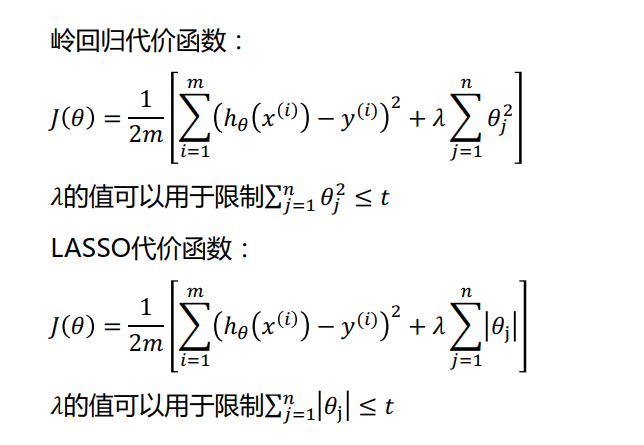LASSO算法的代价函数和岭回归的代价函数都是为了防止过拟合，但是，岭回归算法很难使得模型参数为0，而LASSO算法可以使得模型中的很多参数（对于本数据模型训练影响不大）为0，进而使得模型的特征要素减少
二、sklearn中应有LASSO算法示例代码
import numpy as np
from numpy import genfromtxt
from sklearn import linear_model

# 读取数据
data = genfromtxt(r'longley.csv', delimiter=',')

# 切分数据
x_data = data[1:, 2:]
y_data = data[1:, 1, np.newaxis]

# 训练模型
model = linear_model.LassoCV()
model.fit(x_data, y_data)

# 训练后选择的lasso系数
print(model.alpha_)
# 训练后线性模型参数
print(model.coef_)

# 预测值
print(model.predict(x_data[-2, np.newaxis]))
print(y_data[-2])  # 真实值


三、代码执行结果
20.03464209711722
[0.10206856 0.00409161 0.00354815 0.         0.         0.        ]
[115.6461414]
[115.7]

由上面结果可知，LASSO算法会自动得到一个合适的λ值，并且其训练出的模型中有很多参数为0 ，可以认为参数为0的特征是不重要的特征，可以舍弃，进而简化模型。
四、数据下载
链接：https://pan.baidu.com/s/14xi9nAW4DyY3mWFp_GTb0w
提取码：3kf3


展开全文• 全栈工程师开发手册 (作者：栾鹏)LassoThe Lasso 是估计稀疏系数的线性模型。 它在一些情况下是有用的，因为它倾向于使用具有较少参数值的情况，有效地减少给定解决方案所依赖变量的数量。 因此，Lasso 及其变体是...
全栈工程师开发手册 (作者：栾鹏)LassoThe Lasso 是估计稀疏系数的线性模型。 它在一些情况下是有用的，因为它倾向于使用具有较少参数值的情况，有效地减少给定解决方案所依赖变量的数量。 因此，Lasso 及其变体是压缩感知领域的基础。 在一定条件下，它可以恢复一组非零权重的精确集。在数学公式表达上，它由一个带有ℓ1ℓ1先验的正则项的线性模型组成。 其最小化的目标函数是:minw12nsamples||Xw−y||22+α||w||1minw12nsamples||Xw−y||22+α||w||1lasso 估计解决了加上罚项α||w||1α||w||1的最小二乘法的最小化，其中，αα 是一个常数，||w||1||w||1是参数向量的 ℓ1−normℓ1−norm 范数。Lasso 类的实现使用了 coordinate descent (坐标下降算法)来拟合系数。设置正则化参数scikit-learn 通过交叉验证来公开设置 Lasso中αα 参数的对象: LassoCV 和 LassoLarsCV。 LassoLarsCV 是基于下面解释的 最小角回归 算法。对于具有许多线性回归的高维数据集， LassoCV 最常见。 然而，LassoLarsCV 在寻找 αα 参数值上更具有优势，而且如果样本数量与特征数量相比非常小时，通常 LassoLarsCV 比 LassoCV 要快。与 SVM 的正则化参数的比较αα 和 SVM 的正则化参数CC之间的等式关系是 α=1/Cα=1/C 或者 α=1/(nsamples∗C)α=1/(nsamples∗C)，并依赖于估计器和模型优化的确切的目标函数。主参数设置alpha : float, 可选，默认 1.0。当 alpha 为 0 时算法等同于普通最小二乘法，可通过 Linear Regression 实现，因此不建议将 alpha 设为 0.fit_intercept : boolean是否进行拦截计算(intercept)。若 false，则不计算(比如数据已经经过集中了)。此处不太明白，仿佛与偏度有关。normalize : boolean, 可选, 默认 False若 True，则先 normalize 再 regression。若 fit_intercept 为 false 则忽略此参数。当 regressors 被 normalize 的时候，需要注意超参(hyperparameters)的学习会更稳定，几乎独立于 sample。对于标准化的数据，就不会有此种情况。如果需要标准化数据，请对数据预处理。然后在学习时设置 normalize=False。copy_X : boolean, 可选, 默认 True若 True，则会复制 X；否则可能会被覆盖。precompute : True | False | array-like, 默认=False是否使用预计算的 Gram 矩阵来加速计算。如果设置为 ‘auto’ 则机器决定。Gram 矩阵也可以 pass。对于 sparse input 这个选项永远为 True。max_iter : int, 可选最大循环次数。tol : float, 可选优化容忍度 The tolerance for the optimization: 若更新后小于 tol，优化代码检查优化的 dual gap 并继续直到小于 tol 为止。warm_start : bool, 可选为 True 时, 重复使用上一次学习作为初始化，否则直接清除上次方案。positive : bool, 可选设为 True 时，强制使系数为正。selection : str, 默认 ‘cyclic’若设为 ‘random’, 每次循环会随机更新参数，而按照默认设置则会依次更新。设为随机通常会极大地加速交点(convergence)的产生，尤其是 tol 比 1e-4 大的情况下。random_state : int, RandomState instance, 或者 None (默认值)pseudo random number generator 用来产生随机 feature 进行更新时需要用的seed。仅当 selection 为 random 时才可用。代码实现import numpy as np # 快速操作结构数组的工具import matplotlib.pyplot as plt  # 可视化绘制from sklearn.linear_model import Lasso,LassoCV,LassoLarsCV   # Lasso回归,LassoCV交叉验证实现alpha的选取，LassoLarsCV基于最小角回归交叉验证实现alpha的选取# 样本数据集，第一列为x，第二列为y，在x和y之间建立回归模型data=[[0.067732,3.176513],[0.427810,3.816464],[0.995731,4.550095],[0.738336,4.256571],[0.981083,4.560815],[0.526171,3.929515],[0.378887,3.526170],[0.033859,3.156393],[0.132791,3.110301],[0.138306,3.149813],[0.247809,3.476346],[0.648270,4.119688],[0.731209,4.282233],[0.236833,3.486582],[0.969788,4.655492],[0.607492,3.965162],[0.358622,3.514900],[0.147846,3.125947],[0.637820,4.094115],[0.230372,3.476039],[0.070237,3.210610],[0.067154,3.190612],[0.925577,4.631504],[0.717733,4.295890],[0.015371,3.085028],[0.335070,3.448080],[0.040486,3.167440],[0.212575,3.364266],[0.617218,3.993482],[0.541196,3.891471]]#生成X和y矩阵dataMat = np.array(data)X = dataMat[:,0:1]   # 变量xy = dataMat[:,1]   #变量y# ========Lasso回归========model = Lasso(alpha=0.01)  # 调节alpha可以实现对拟合的程度# model = LassoCV()  # LassoCV自动调节alpha可以实现选择最佳的alpha。# model = LassoLarsCV()  # LassoLarsCV自动调节alpha可以实现选择最佳的alphamodel.fit(X, y)   # 线性回归建模print('系数矩阵:n',model.coef_)print('线性回归模型:n',model)# print('最佳的alpha：',model.alpha_)  # 只有在使用LassoCV、LassoLarsCV时才有效# 使用模型预测predicted = model.predict(X)# 绘制散点图 参数：x横轴 y纵轴plt.scatter(X, y, marker='x')plt.plot(X, predicted,c='r')# 绘制x轴和y轴坐标plt.xlabel("x")plt.ylabel("y")# 显示图形plt.show()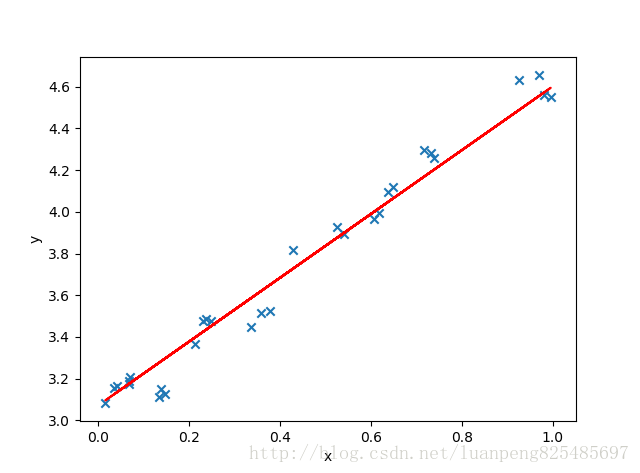多任务回归MultiTaskLasso 是一个估计多元回归稀疏系数的线性模型： yy是一个 (nsamples,ntasks)(nsamples,ntasks) 的二维数组，其约束条件和其他回归问题(也称为任务)是一样的，都是所选的特征值。下图比较了通过使用简单的 Lasso 或 MultiTaskLasso 得到的 W 中非零的位置。 Lasso 估计产生分散的非零值，而 MultiTaskLasso 的一整列都是非零的。在数学上，它由一个线性模型组成，以混合的 ℓ1ℓ2ℓ1ℓ2作为正则化器进行训练。目标函数最小化是：minw12nsamples||XW−Y||2Fro+α||W||21minw12nsamples|版权声明：本文来源CSDN，感谢博主原创文章，遵循 CC 4.0 by-sa 版权协议，转载请附上原文出处链接和本声明。原文链接：https://blog.csdn.net/luanpeng825485697/article/details/79829926站方申明：本站部分内容来自社区用户分享，若涉及侵权，请联系站方删除。发表于 2020-03-01 19:58阅读 ( 190 )0 推荐收藏
展开全文• ## sklearn中的LASSO

千次阅读 2019-02-27 18:40:31
LASSO import numpy as np import matplotlib.pyplot as plt np.random.seed(42) x = np.random.uniform(-3.0, 3.0, size=100) X = x.reshape(-1, 1) y = 0.5 * x + 3 + np.random.normal(0, 1, size=100) plt.sca...
LASSO
import numpy as np
import matplotlib.pyplot as plt

np.random.seed(42)
x = np.random.uniform(-3.0, 3.0, size=100)
X = x.reshape(-1, 1)
y = 0.5 * x + 3 + np.random.normal(0, 1, size=100)

plt.scatter(x, y)
plt.show()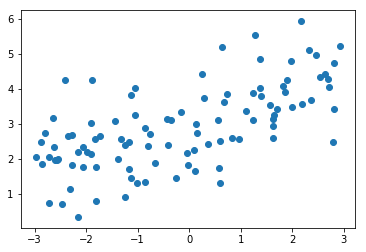from sklearn.model_selection import train_test_split

np.random.seed(666)
X_train, X_test, y_train, y_test = train_test_split(X, y)

from sklearn.pipeline import Pipeline
from sklearn.preprocessing import PolynomialFeatures
from sklearn.preprocessing import StandardScaler
from sklearn.linear_model import LinearRegression

def PolynomialRegression(degree):
return Pipeline([
("poly", PolynomialFeatures(degree=degree)),
("std_scaler", StandardScaler()),
("lin_reg", LinearRegression())
])

from sklearn.metrics import mean_squared_error

poly_reg = PolynomialRegression(degree=20)
poly_reg.fit(X_train, y_train)

y_predict = poly_reg.predict(X_test)
mean_squared_error(y_test, y_predict)

167.94010867293571

def plot_model(model):
X_plot = np.linspace(-3, 3, 100).reshape(100, 1)
y_plot = model.predict(X_plot)

plt.scatter(x, y)
plt.plot(X_plot[:,0], y_plot, color='r')
plt.axis([-3, 3, 0, 6])
plt.show()

plot_model(poly_reg)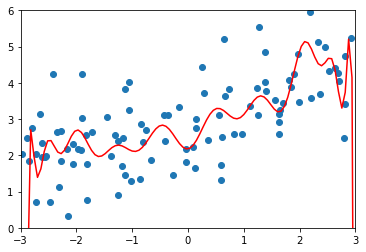from sklearn.linear_model import Lasso

def LassoRegression(degree, alpha):
return Pipeline([
("poly", PolynomialFeatures(degree=degree)),
("std_scaler", StandardScaler()),
("lasso_reg", Lasso(alpha=alpha))
])

lasso1_reg = LassoRegression(20, 0.01)
lasso1_reg.fit(X_train, y_train)

y1_predict = lasso1_reg.predict(X_test)
mean_squared_error(y_test, y1_predict)

1.1496080843259966

plot_model(lasso1_reg)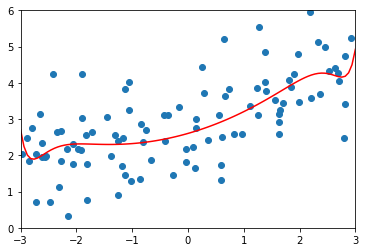lasso2_reg = LassoRegression(20, 0.1)
lasso2_reg.fit(X_train, y_train)

y2_predict = lasso2_reg.predict(X_test)
mean_squared_error(y_test, y2_predict)

1.1213911351818648

plot_model(lasso2_reg)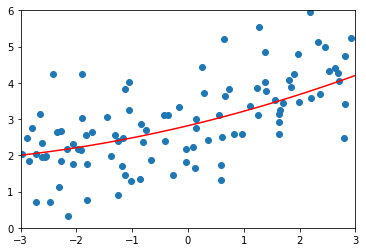lasso3_reg = LassoRegression(20, 1)
lasso3_reg.fit(X_train, y_train)

y3_predict = lasso3_reg.predict(X_test)
mean_squared_error(y_test, y3_predict)

1.8408939659515595

plot_model(lasso3_reg)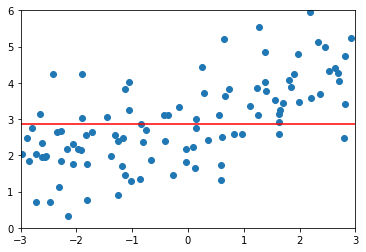展开全文• ## sklearn中的Lasso函数

千次阅读 2018-05-23 20:31:00
Lasso(alpha=1.0, fit_intercept=True, normalize=False, precompute=False, copy_X=True, max_iter=1000, tol=1e-4, warm_start=False, positive=False, random_state=None, selection=’cyclic’) 类型：  ...
语法：

Lasso(alpha=1.0, fit_intercept=True, normalize=False, precompute=False, copy_X=True, max_iter=1000, tol=1e-4, warm_start=False, positive=False, random_state=None, selection=’cyclic’)

类型：

在sklearn.linear_model.coordinate_descent中出现，Lasso类，继承自ElasticNet。使用L1作为正则化器训练的线性模型（即套索）。

Lasso的最优目标函数是：
12⋅n_samples||y−Xw||22+α||w||1$\frac{1}{2\cdot n\mathrm{_}samples}||y-Xw|{|}_{2}^{2}+\alpha ||w|{|}_{1}$
\frac 1 {2\cdot n\_samples} ||y-Xw||^2_2+\alpha||w||_1

从技术上讲，Lasso模型优化了与l1_ratio=1.0$l1\mathrm{_}ratio=1.0$$l1\_ratio=1.0$（没有L2惩罚）的弹性网相同的目标函数。

在用户指导手册中查阅更多。

输入参数：

参数名：alpha
类型： float, optional *
说明：与L1项相乘的常数。默认为1.0。α=1$\alpha =1$$\alpha=1$等价于一个普通最小平方，由线性回归对象求解。由于数值原因，不建议α$\alpha$$\alpha$设置为0 的lasso对象。如果这样设置了，应该使用线性回归对象。

参数名：fit_intercept
类型： boolean
说明：是否计算该模型的截距。如果设置为False，将不会在计算中使用截距（比如，预处理数据已经中心化）

参数名：normalize
类型： boolean, optional, default False
说明：当fit_intercept设置为False时，该参数将会被忽略。如果为True，则回归前，回归变量X将会进行归一化，减去均值，然后除以L2范数。如果想要标准化，请在评估器（normalize参数为False）调用fit方法前调用sklean.preprocessing.StandardScaler。

参数名：precompute
类型：True | False | array-like, default=False
说明：是否使用预计算的Gram矩阵来加速计算。如果设置为’auto’让我们来决定。Gram矩阵也可以作为参数传递。对于稀疏输入，这个选项总是正确的，以保持稀疏性。

参数名：copy_X
类型：boolean, optional, default True
说明：如果是True，x将被复制，否则，有可能被覆盖。

参数名：max_iter
类型： int, optional
说明：最大迭代次数。

参数名：tol
类型：float, optional
说明：优化器的公差。如果更新值小于tol，优化器代码检查双差距的最佳性，并继续直到它小于tol。

参数名：warm_start
类型： bool, optional
说明：当设置为True时，重用之前调用的解决方案作为初始化，否则，只需要删除前面的解决方案

参数名：positive
类型：bool, optional
说明：当设为真时，系数为正。

参数名：random_state
类型：int, RandomState instance or None, optional, default None
说明：在对数据进行变换时使用伪随机数生成器的种子。如果int, random_state是随机数生成器使用的种子;如果随机状态实例，random_state是随机数发生器;如果没有，随机数生成器就是np.random使用的随机状态实例。当选择为 ’ random ‘时使用。

参数名：selection
类型：str, default ‘cyclic’
说明：如果设置为“random”，则每一次迭代都会更新一个随机系数，而不是在默认情况下按顺序循环。这(设置为“random”)通常会导致更快的收敛速度，尤其是当tol大于1e-4时。

属性

参数名：coef_
类型：array, shape (n_features,) | (n_targets, n_features)
说明：参数向量(损失函数表达式中的w$w$$w$)

参数名：sparse_coef_
类型：scipy.sparse matrix, shape (n_features, 1) | (n_targets, n_features)
说明：sparse_coef_ 是从coef_ 导出的只读属性

参数名：intercept_
类型：float | array, shape (n_targets,)
说明：决策函数中的独立项，即截距

参数名：n_iter_
类型： int | array-like, shape (n_targets,)
说明：由坐标下降求解器运行的，达到指定公差的迭代次数。

示例

from sklearn import linear_model
clf = linear_model.Lasso(alpha=0.1)
clf.fit([[0,0], [1, 1], [2, 2]], [0, 1, 2])
print(clf.coef_)
# [ 0.85  0.  ]
print(clf.intercept_)
# 0.15

参阅

lars_path ，lasso_path， LassoLars， LassoCV ，LassoLarsCV

sklearn.decomposition.sparse_encode

笔记

用于拟合模型的算法是坐标下降法。

为了避免不必要的内存复制，应该将fit方法的X参数直接作为一个Fortran连续numpy数组传递。 
展开全文• 本文主要讲一些sklearn中回归模型的使用，如果需要了解相关理论，请查阅：【线性回归】面向新手的基础知识 线性回归 引入 from sklearn.linear_model import LinearRegression # 默认参数如下： LinearRegression...机器学习 算法 python
• ## sklearn-1.1.3.Lasso

千次阅读 2018-06-04 21:44:31
1.1.3套索套索回归是一个稀疏系数的线性回归。当参数值较少的时候，它很有用，有效的减少...例如，这组数据是从CT获得的。没有事先样本的相关信息，重构图片所需的投影数量是图像的线性尺寸I的量级（以像素为单位...
• # 基于糖尿病的数据集，基于Lasso计算特则的相关系数路径，Lasso中对L1正则项进行优化 # 图示每一个颜色代表了一个特征值的相关系数向量 # 本例使用Lasso模型基于数据集进行优化，得到不同超参数值的情况下，...
• 文章目录一、linear_model.LassoCV()♦ 正则化路径 regularization path♦ linear_model.LassoCV...之前提到过,由于 Lasso对正则化系数的变动过于敏感,因此我们往往让α在很小的空间变动。这个小空间小到超乎人们的..机器学习
• sklearn中拥有有非常庞大的线性回归模型家族，采用各种算法用以解决各类线性回归问题。不同的线性回归模型的参数设置、模型方法和调参策略并不一样，本文并不具体介绍每个模型的具体接口和使用事项，仅简单梳理下...线性回归